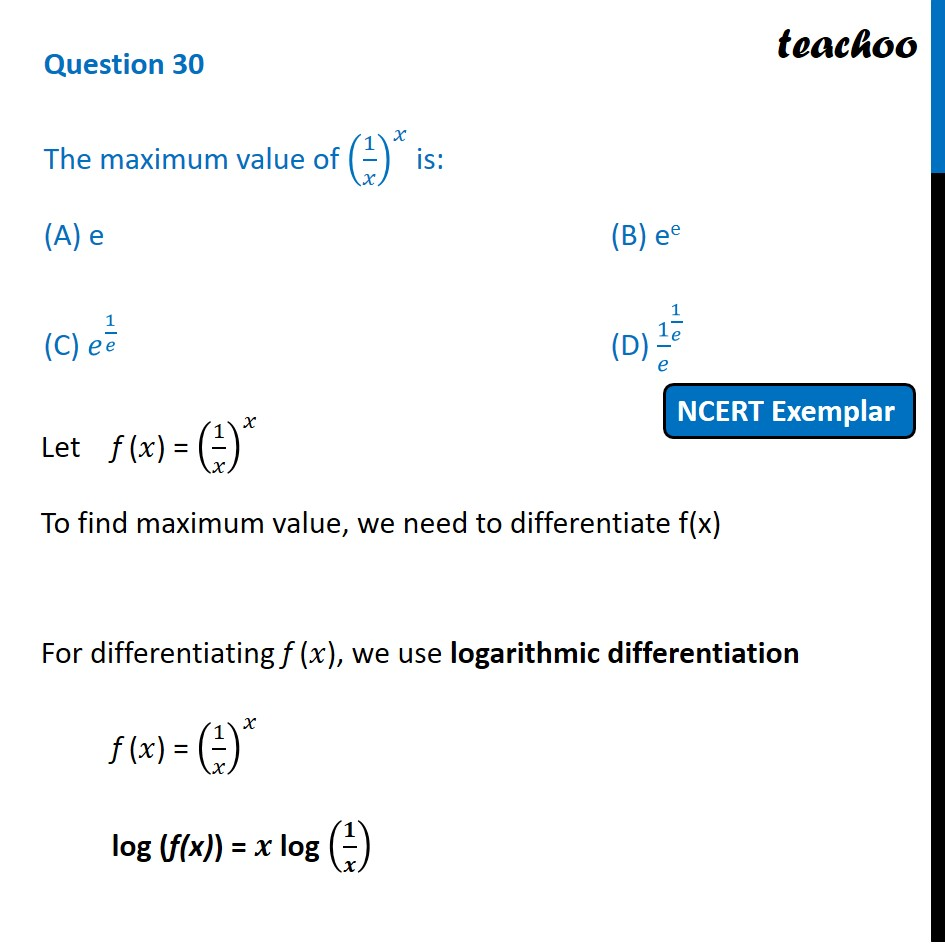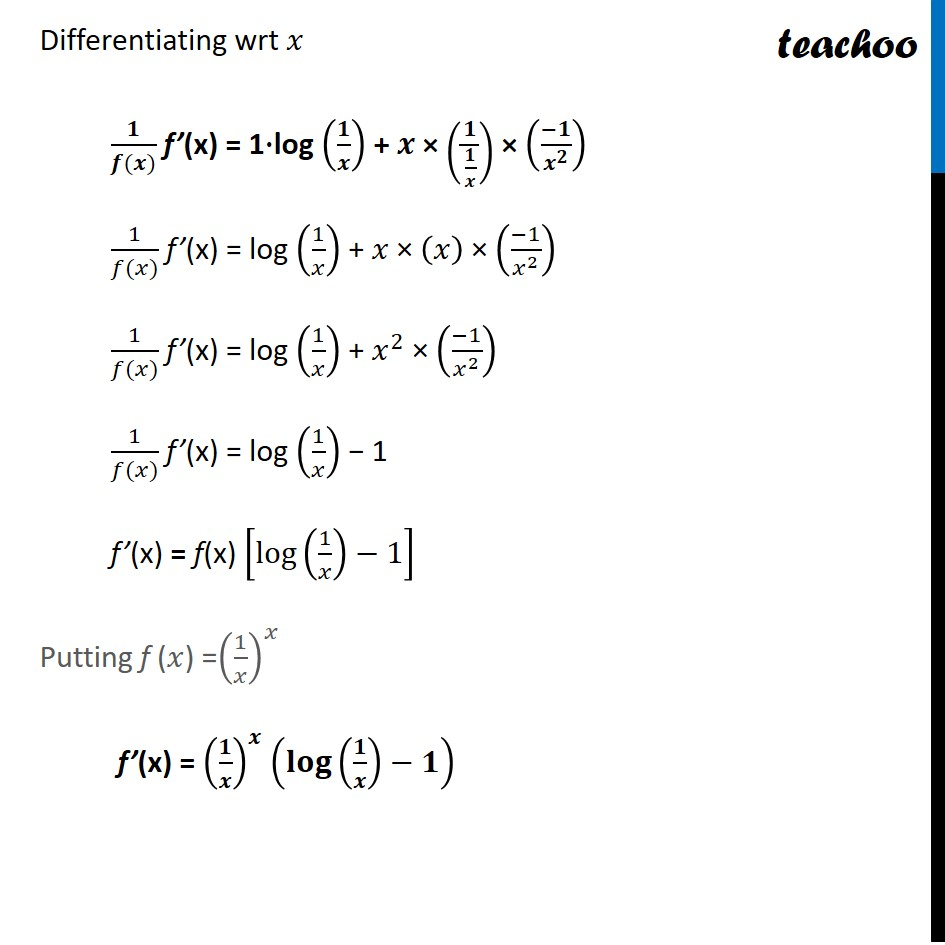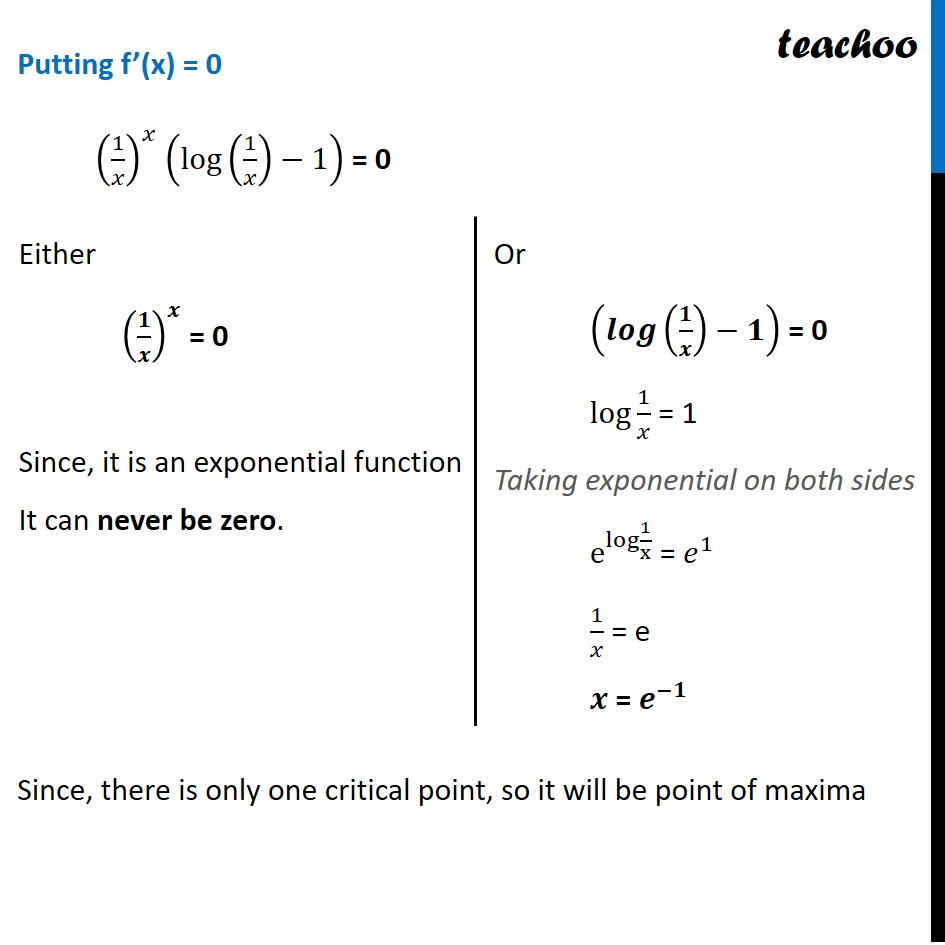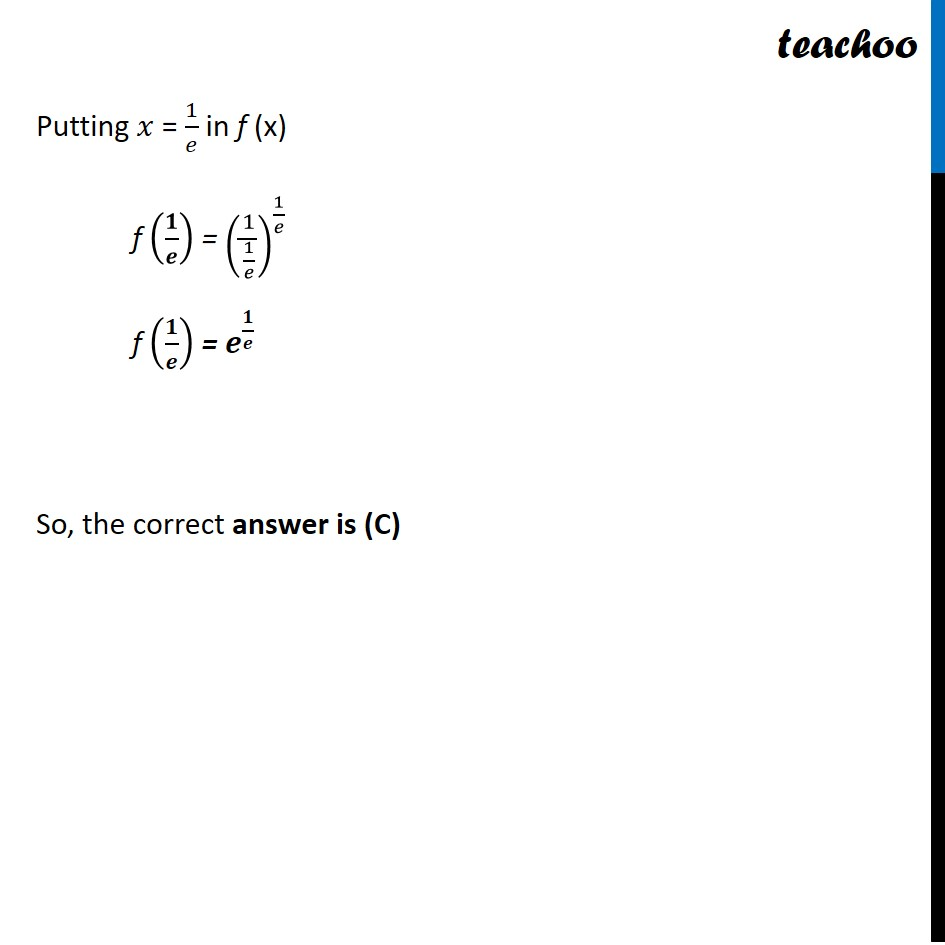NCERT Exemplar - MCQs

Chapter 6 Class 12 Application of Derivatives
Serial order wise

## (C) e (1/e)                       (D) 1/e (1/e)Learn in your speed, with individual attention - Teachoo Maths 1-on-1 Class

### Transcript

Question 17 The maximum value of (1/𝑥)^𝑥 is: (A) e (B) ee (C) 𝑒^(1/𝑒) (D) 〖1/𝑒〗^(1/𝑒) Let f (𝑥) = (1/𝑥)^𝑥 To find maximum value, we need to differentiate f(x) For differentiating f (𝑥), we use logarithmic differentiation f (𝑥) = (1/𝑥)^𝑥 log (f(x)) = 𝒙 log (𝟏/𝒙) Differentiating wrt 𝑥 𝟏/(𝒇(𝒙)) f’(x) = 1∙log (𝟏/𝒙) + 𝒙 × (𝟏/(𝟏/𝒙)) × ((−𝟏)/𝒙^𝟐 ) 1/(𝑓(𝑥)) f’(x) = log (1/𝑥) + 𝑥 × (𝑥) × ((−1)/𝑥^2 ) 1/(𝑓(𝑥)) f’(x) = log (1/𝑥) + 𝑥^2 × ((−1)/𝑥^2 ) 1/(𝑓(𝑥)) f’(x) = log (1/𝑥) − 1 f’(x) = f(x) [log⁡〖(1/𝑥)−1〗 ] Putting f (𝑥) =(1/𝑥)^𝑥 f’(x) = (𝟏/𝒙)^𝒙 (𝐥𝐨𝐠⁡〖(𝟏/𝒙)−𝟏〗 ) Putting f’(x) = 0 (1/𝑥)^𝑥 (log⁡〖(1/𝑥)−1〗 ) = 0 Since, there is only one critical point, so it will be point of maxima Either (𝟏/𝒙)^𝒙 = 0 Since, it is an exponential function It can never be zero. Or (𝒍𝒐𝒈⁡〖(𝟏/𝒙)−𝟏〗 ) = 0 log 1/𝑥 = 1 Taking exponential on both sides e^log⁡〖1/x〗 = 𝑒^1 1/𝑥 = e 𝒙 = 𝒆^(−𝟏) Putting 𝑥 = 1/𝑒 in f (x) f (𝟏/𝒆) = (1/(1/𝑒))^(1/𝑒) f (𝟏/𝒆) = 𝒆^(𝟏/𝒆) So, the correct answer is (C)